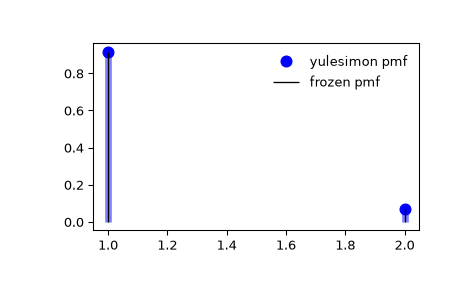# scipy.stats.yulesimon¶

scipy.stats.yulesimon = <scipy.stats._discrete_distns.yulesimon_gen object>[source]

A Yule-Simon discrete random variable.

As an instance of the rv_discrete class, yulesimon object inherits from it a collection of generic methods (see below for the full list), and completes them with details specific for this particular distribution.

Notes

The probability mass function for the yulesimon is:

$f(k) = \alpha B(k, \alpha+1)$

for $$k=1,2,3,...$$, where $$\alpha>0$$. Here $$B$$ refers to the scipy.special.beta function.

The sampling of random variates is based on pg 553, Section 6.3 of . Our notation maps to the referenced logic via $$\alpha=a-1$$.

For details see the wikipedia entry .

References

1

Devroye, Luc. “Non-uniform Random Variate Generation”, (1986) Springer, New York.

2

https://en.wikipedia.org/wiki/Yule-Simon_distribution

The probability mass function above is defined in the “standardized” form. To shift distribution use the loc parameter. Specifically, yulesimon.pmf(k, alpha, loc) is identically equivalent to yulesimon.pmf(k - loc, alpha).

Examples

>>> from scipy.stats import yulesimon
>>> import matplotlib.pyplot as plt
>>> fig, ax = plt.subplots(1, 1)


Calculate the first four moments:

>>> alpha = 11
>>> mean, var, skew, kurt = yulesimon.stats(alpha, moments='mvsk')


Display the probability mass function (pmf):

>>> x = np.arange(yulesimon.ppf(0.01, alpha),
...               yulesimon.ppf(0.99, alpha))
>>> ax.plot(x, yulesimon.pmf(x, alpha), 'bo', ms=8, label='yulesimon pmf')
>>> ax.vlines(x, 0, yulesimon.pmf(x, alpha), colors='b', lw=5, alpha=0.5)


Alternatively, the distribution object can be called (as a function) to fix the shape and location. This returns a “frozen” RV object holding the given parameters fixed.

Freeze the distribution and display the frozen pmf:

>>> rv = yulesimon(alpha)
>>> ax.vlines(x, 0, rv.pmf(x), colors='k', linestyles='-', lw=1,
...         label='frozen pmf')
>>> ax.legend(loc='best', frameon=False)
>>> plt.show()Check accuracy of cdf and ppf:

>>> prob = yulesimon.cdf(x, alpha)
>>> np.allclose(x, yulesimon.ppf(prob, alpha))
True


Generate random numbers:

>>> r = yulesimon.rvs(alpha, size=1000)


Methods

 rvs(alpha, loc=0, size=1, random_state=None) Random variates. pmf(k, alpha, loc=0) Probability mass function. logpmf(k, alpha, loc=0) Log of the probability mass function. cdf(k, alpha, loc=0) Cumulative distribution function. logcdf(k, alpha, loc=0) Log of the cumulative distribution function. sf(k, alpha, loc=0) Survival function (also defined as 1 - cdf, but sf is sometimes more accurate). logsf(k, alpha, loc=0) Log of the survival function. ppf(q, alpha, loc=0) Percent point function (inverse of cdf — percentiles). isf(q, alpha, loc=0) Inverse survival function (inverse of sf). stats(alpha, loc=0, moments=’mv’) Mean(‘m’), variance(‘v’), skew(‘s’), and/or kurtosis(‘k’). entropy(alpha, loc=0) (Differential) entropy of the RV. expect(func, args=(alpha,), loc=0, lb=None, ub=None, conditional=False) Expected value of a function (of one argument) with respect to the distribution. median(alpha, loc=0) Median of the distribution. mean(alpha, loc=0) Mean of the distribution. var(alpha, loc=0) Variance of the distribution. std(alpha, loc=0) Standard deviation of the distribution. interval(alpha, alpha, loc=0) Endpoints of the range that contains fraction alpha [0, 1] of the distribution